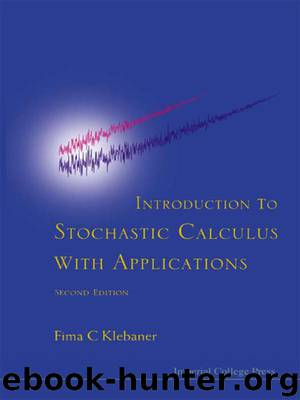# Introduction to Stochastic Calculus With Applications: Second Edition by Fima C KlebanerAuthor:Fima C Klebaner
Language: eng
Format: epub
Publisher: Imperial College Press

Thus we have shown that when p ≥ 1/2 any positive state will be reached from 0 in a finite time, but when p = 1/2 the average time for it to happen is infinite.

The results obtained above are known as transience (p ≠ 1/2) and recurrence (p = 1/2) of the Random Walk, and are usually obtained by Markov Chains Theory.

Example 7.9: (Optional stopping of discrete time martingales)

Let M(t) be a discrete time martingale and be a stopping time such that E|M()| < ∞.

1. If E < ∞ and |M(t + 1) − M(t)| ≤ K, then EM() = EM(0).

2. If E < ∞ and E(|M(t + 1) − M(t)||Ft) ≤ K, then EM() = EM(0).

PROOF: We prove the first statement.

M(t) = M(0)|+ (M(i+1) − M(i)). This together with the bound on increments gives

M(t) ≤ |M(0)| +|M(i + 1) − M(i)| ≤ |M(0)| + Kt.

Take for simplicity non-random M(0) Then

EM(t)I( > t) ≤ |M(0)|P( > t) + KtP( > t).

The last term converges to zero, tP( > t) ≤ E( I( > t)) → 0, by dominated convergence due to E( ) < ∞. Thus condition (7.8) holds, and the result follows. The proof of the second statement is similar and is left as an exercise.